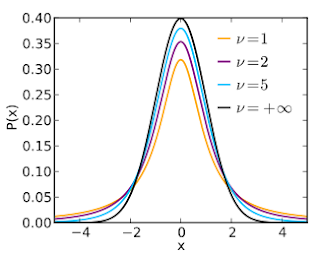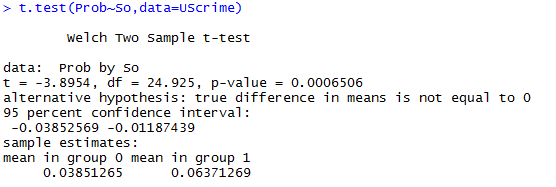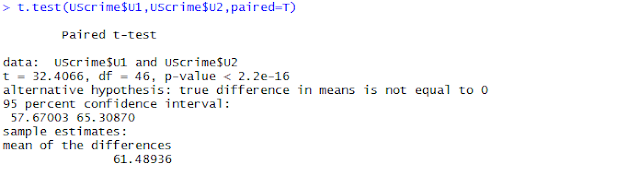## What is difference between Correlation and Covariance?Correlation is standardized form of covariance. That means you can compare correlation of two data sets having different units.

You can not compare covariance of two data sets that have different units.

Now, how to find correlation and covariance in R?

Two functions –

• cor() for calculating correlations
• cov() for calculating covariance

Syntax for both of them is same

• cor(x,y,use,method)

Where x and y can be a variable/matrix or data frame.

“use” is basically to handle missing values. It can have following options

• “everything” (default)- Everything will be included and if the datasets have NA values then correlation will also have a corresponding NAs
• “all.obs” – If NAs is/are present, an error will be returned
• “complete.obs”- list wise deletion of NA values is done
• “pairwise.complete.obs”-pairwise deletion of NA values is done

method specifies which correlation we want to calculate

• Pearson
• Spearman
• Kendell

Most of the times we would be using Pearson only, which is a parametric correlation, that it takes assumptions. Which are – the data sets are normally distributed and they are linear.

Spearman is used mostly in case of ordinal data, when the data sets have a monotonic relationship rather than the linear one. This one is non parametric, that is it takes no assumptions.

But, the case is not solved yet. Just by knowing the coefficient of correlation we can’t say that two datasets are having strong/weak/no relationship. The result can also be because of the choice of sample and thus the results can simply be because of chance. Thus, we also need to find the significance of correlation.

To find significance of a result to reject the NULL hypothesis we usually use the p-value. We reject the NULL hypothesis when p-value is less than the level of significance.

So, if the coefficient of correlation is high and the p-value is more than the level of significance, we can’t comment on the relationship as it simply means that the results are because of chance and a different sample of same population might produce a different result.

In comes “Hmisc” package

it has a function to solve our purpose, the rcorr() function. This function provides both the strength of correlation as well the corresponding p-values.

This one also takes the same arguments. The input to this function is always a matrix, thus you have to use the as.matrix() function on inputs that are not of the required type, also make sure that you put use=”pairwise.complete.cases”.

The methods can be any of Pearson or Spearman

Again the “Hmisc” package

But, you know what would be really interesting? Plotting the correlations.

For this we need the corrgram() function of corrgram package package.

Lets plot the correlation of all the variables of mtcars data set.

>library(corrgram)

>corrgram(mtcars)The color scale goes from red to blue. Intensity of red obviously indicating the negative intensity and intensity of blue indicating the positive intensity

In the above plot we can clearly see that mileage per gallon has negative correlation with cyllinders, displacement, horse power and weight.

Sweet, now my post has a picture.

Best,
Sanmeet,
Founder, DataVinci
[testimonials_cycle theme=”default_style” count=”-1″ order_by=”date” order=”ASC” show_title=”0″ use_excerpt=”0″ show_thumbs=”1″ show_date=”1″ show_other=”1″ hide_view_more=”0″ output_schema_markup=”1″ show_rating=”stars” testimonials_per_slide=”1″ transition=”fade” timer=”5000″ pause_on_hover=”true” auto_height=”container” show_pager_icons=”1″ prev_next=”1″ display_pagers_above=”0″ paused=”0″]

## What is t-test? How to do t-test in R?

Alright, first thing that makes me curious about Student’s t-test is why it is called so. The history is somewhat interesting.

First, why the “Student”?

“Student” is actually pseudonym or pen name of the author, Mr. William Sealy Gosset. He used a pen name because at the time he came up the concept he was not allowed to publish stuff under his own name by his employer. Some company with bad GTPW ratings it seems

Here is the dude –Second, why the “T”?

“t-test” comes from “t-distribution” which in turn comes from “t-statistic” which in turn stands for “test – statistic” which in turn is short for “hypothesis test statistic”

So, brother, what is hypothesis test statistic man?

Hint1: It is used to test some hypothesis!

Hint2: hypothesis to verify any existence of difference between two data sets!

Yes, you smart ass, you got it right! t-statistic is used to perform hypothesis testing around sample mean. But, wait a second. There are plethora of methods out there to test hypothesis around sample means, but when will ya prefer t-test?

Good Question!

The fundamental requirement for using the t-test is that your population should be normally distributed. If you are not sure about the distribution of the population, you can still use the t-distribution if your sample is large enough to satisfy the central limit theorem. If the case is not so, go for some other test like Mann-Whittney U-test.

Second, you will use t-test when the standard deviation of the “Population” is not known. There are like thousands of posts out there who claim that t-statistic should be used when the sample size is less than equal to 30. But, this is not true. So, reiterating : Use a t-test when the standard deviation of the population is not know.

Now, what is t-statistic?

Expressing it in words:

Lets understand t-statistic for a small sample of variable X. The mean of the population is M and mean of sample is Ms.

t-statistic is a ratio of variance between groups to variance within the groups.

So, the formula –

t-statistic = (Ms – M)/SE

Moving forward, lets understand the t-distribution –

Now, as we can see above t-statistic is dependent on standard error, which in turn is dependent on the size of the sample. And because different samples will have different standard errors and different degrees of freedom, there will be multiple t-distributions of the sample variable based on the size of the sample.

How does t-distribution look?

t-distribution is also a bell shaped curve, but it has more area in the tails as compared to area in the tails of normal random distribution of a population. As the size of the sample increases the shape of t-distribution evolves to match the shape of the normal random population distribution and at a sample size of infinity, t-distribution is exactly like the normal random population distribution.Now, how do you do a t-test?

Any statistical test will have some confidence interval. For a given confidence interval and degree of freedom, you will use the t-statistic to calculate the p-value using which you will accept or reject the null hypothesis.

p-value on a t-distribution curve for a particular t-statistic will be area of the curve to the right of that t-statistic

The degree of freedom for a sample of size n is n-1.

Before explaining the steps, let me caress upon one tail/ two tail tests. By default all tests are two tail, which assumes that your sample mean can either be less or more than the population mean, that is you test in both the directions, that’s why 2 tail.

So, the null hypothesis of a two tail test is that there is no difference between sample mean and population mean.

When you take a single tail test, then depending upon whether it is upper tail or lower tail, you check for only one case. If its lower tail, you test whether the sample mean is less than or equal to population mean or not. And if it is upper tail, you test whether the sample mean is greater than or equal to the population mean or not.

Read again and assimilate.

So, the null hypothesis of a one tail test, based on the choice of tail, would be either the sample mean being greater than population mean or it being less than the population mean.

Alrighty!

The complement of confidence interval is level of significance. For example, if you have a 95% confidence interval then you level of significance is 5%. Now, depending on whether you take one tail or two tail test, you will use the significance level.

Just to clarify what a 5% level of significance indicates – You can erroneously reject the null hypothesis in probably 5% cases. That is 95% of the times you will not make an error.

Now, finally, the steps –

Case #1 – H0: Sample mean is more than population mean

1. Calculate the t-statistic using the formula

2. For a given t-statistic using software find out the corresponding p value

As mentioned p value gives area to the right of the test statistic on t-distribution curve. That area corresponds to probability of type 1 error

3. Compare p value with level of significance

4. if p value is less than level of significance reject the null hypothesis

Case #2 – Sample mean is less than population mean

Step 1 and 2 are same as above

3. Subtract the p-value from 1

This gives the area to the left of t-statistic on the t-distribution curve

Case # 3 – Sample mean is not equal to population mean, it can be either greater or lesser

Step 1 and 2 are same

3. Double the p-value to accommodate both the cases

4. Compare the doubled value with level of significance

Easy peasy!

Now, ladies and gentlemen lets see how to do a t-test in “R”

Lets start with the independent t-test. Independent t-test is used to prove/disprove similarity between samples of data taken from independent populations.

To understand this, lets the “UScrime” data set in the “MASS” packageHere, the variable SO tells whether the data point is for southern state or not. Prob tells about the probability of imprisonment.

We want to find whether the probability of imprisonment any way affected by the state.

Our Null Hypothesis is that there is no impact on imprisonment based on the state.

The function to run the t-test in R is t.test. It takes either of the following syntax :

t.test(y~x,data)

where x is a dichotomous variable and y is numeric

or

t.test(y1,y2)

where y1 and y2 both are numeric

By default a 2-tail test is assumed. If you want to make it a one tail, then you need to add another argument – alternative=”less” or alternative=”greater”

Lets run the test –Here the p-value is very less than the significance level of 0.05. That is there is indeed a difference between the data from South and non south states. And the probability of this difference being just because of chance is less than 5%.

Next, Dependent t-tests

In case of dependent t-tests, the two populations are not independent of each other, that is they affect each other. Lets take case of unemployment, for limited number of jobs, employment of younger people will affect the employment of some what older people and vice versa

To run a dependent t test in R we simply need to add “paired = T” argument along with the data inputs in the t.test() function.

t.test(y1,y2,paired=T)

where y1 and y2 are numeric data

Ex-With this more or less we should be good with t-test.

Best,

Sanmeet

Founder, DataVinci[testimonials_cycle theme=”default_style” count=”-1″ order_by=”date” order=”ASC” show_title=”0″ use_excerpt=”0″ show_thumbs=”1″ show_date=”1″ show_other=”1″ hide_view_more=”0″ output_schema_markup=”1″ show_rating=”stars” testimonials_per_slide=”1″ transition=”fade” timer=”5000″ pause_on_hover=”true” auto_height=”container” show_pager_icons=”1″ prev_next=”1″ display_pagers_above=”0″ paused=”0″]

## What’s the Difference Between a CPU and a GPU?The CPU (central processing unit) has often been called the brains of the PC. But increasingly, that brain is being enhanced by another part of the PC – the GPU (graphics processing unit), which is its soul.

Cpu-vs-gpu All PCs have chips that render the display images to monitors. But not all these chips are created equal. Intel’s integrated graphics controller provides basic graphics that can display only productivity applications like Microsoft PowerPoint, low-resolution video and basic games.

The GPU is in a class by itself – it goes far beyond basic graphics controller functions, and is a programmable and powerful computational device in its own right.

## What Is a GPU?

The GPU’s advanced capabilities were originally used primarily for 3D game rendering. But now those capabilities are being harnessed more broadly to accelerate computational workloads in areas such as financial modeling, cutting-edge scientific research and oil and gas exploration.

In a recent BusinessWeek article, Insight64 principal analyst Nathan Brookwood described the unique capabilities of the GPU this way: “GPUs are optimized for taking huge batches of data and performing the same operation over and over very quickly, unlike PC microprocessors, which tend to skip all over the place.”

Architecturally, the CPU is composed of just few cores with lots of cache memory that can handle a few software threads at a time. In contrast, a GPU is composed of hundreds of cores that can handle thousands of threads simultaneously. The ability of a GPU with 100+ cores to process thousands of threads can accelerate some software by 100x over a CPU alone. What’s more, the GPU achieves this acceleration while being more power- and cost-efficient than a CPU.

## GPU-Accelerated Computing Goes Mainstream

GPU-accelerated computing has now grown into a mainstream movement supported by the latest operating systems from Apple (with OpenCL) and Microsoft (using DirectCompute). The reason for the wide and mainstream acceptance is that the GPU is a computational powerhouse, and its capabilities are growing faster than those of the x86 CPU.

In today’s PC, the GPU can now take on many multimedia tasks, such as accelerating Adobe Flash video, transcoding (translating) video between different formats, image recognition, virus pattern matching and others. More and more, the really hard problems to solve are those that have an inherent parallel nature – video processing, image analysis, signal processing.

The combination of a CPU with a GPU can deliver the best value of system performance, price, and power.

## Here is a table summarizing the differences further :## Here is brilliant YouTube Video explaining the two units :

Hope you liked this post!

Best,
Team DataVinci## How to track items without attributes through Tag Manager

Jquery(function(){

\$(“a”).addClass(function(index){

\$(this).addClass(“item”+ index);

});

\$(“div”).addClass(function(currentClass,index){

if(currentClass===”dummy”){

return “item” + index;

}

});

});

## How to Track AJAX site in Adobe DTM (Dynamic Tag Manager)

To Track Ajax based websites using Adobe DTM we need to use the Direct Call Rules.

Essentially, these are situations in which the content of the page changes without any change in URL or HTML elements. For example, a multi step form where in different steps of a form appear based on user interaction without any change in page URL

To implement Direct Call Rule, you will need support from the Dev team

The Dev team needs to call the function “_satellite.track(“Name of action”) ” in a JavaScript after successful rendering of the required user experience. Please note “Name of action” is just an example, it can be anything based on the business case.

Then, you need to mention the Direct Call String in the “String” field of Direct Call Rule condition in the DTM interface. In our example, string will be Name of action

Please note, _satellite.track only notifies DTM about existence of a condition that needs to be tracked. It does not tell what to track

_satellite.track tells DTM when to track

_satellite.track doesn’t tell DTM what to track

We need to configure the Adobe Analytics settings within the Direct Call Rule in DTM interface to tell DTM what to track

For example eVar1=”Step 1″

We can also use Data Elements to do this.

Now, what if we want to capture something which is not pre populated within the Data Layer?

Under such condition we need to dynamically create the Data Elements as well.

We can create Data Elements dynamically by using the _satellite.setVar(“Name of Data Element”,Value) function. This function also needs to be passed by the developer.

So, lets say user selects a particular option from drop down in the form and then completes step 1 of the form such that the page URL does not change. The developer will need to pass something like this in the JavaScript function.

<script>

var FormElement = JQuerycode to capture dropdown option;

_satellite.setVar(“Form Dropdown selection”,FormElement);

_satellite.track(“Form Step 1 complete”)

</script>

Once, the above has been configured on the page, we need to go to Direct Call rules, under condition we need to write Form Step 1 Complete and then we can configure any of the custom variables by typing in %Form Dropdown selection% in the value field for that variable.

Kindly leave your comment below.

If you would like to receive our latest posts kindly subscribe to the blog

Best,

Sanmeet Singh Walia

Founder, DataVinci